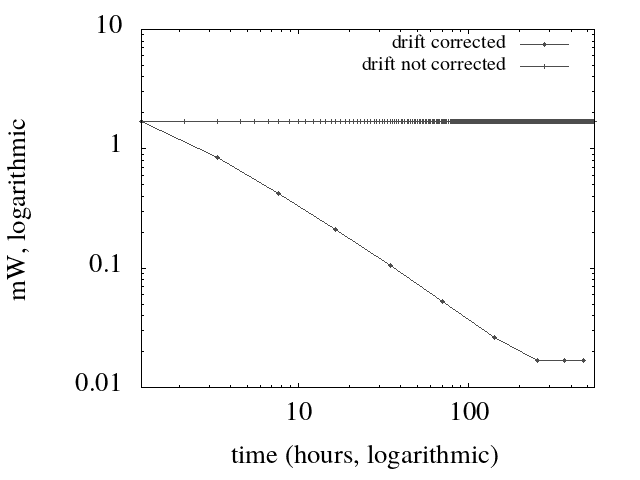# Power-Aware Synchronization of a Software Defined Clock

Like any other computational activity, the maintenance of a local instance of a shared time reference requires a certain amount of power: when available, a shared time reference significantly simplifies, for instance, coordination tasks and logging. In systems where power saving is an issue, like in sensors that operate on batteries or harvesting energy from the environment, it is of vital to understand how much computation and communication is needed to implement such functionality, and to effectively minimize its impact, in order to resolve the trade-off whether to implement or not such feature. A design that target an optimal use of power resources is challenging, since the computational resources are extremely limited. In this entry we give an overview of the main issues, and indicate the fundamental concepts of the solution we propose.

Table of Content [Hide]

Measuring the time is a challenging matter, yet we need to acquire the knowledge of this ephemeral quantity in order to realize a number of tasks. In computer science the sharing of a common time reference in a system of autonomous components is a well studied problem, and a number of protocols have been standardized to exchange time information. However, when we apply such protocols to sensor networks we notice a couple of issues which are negligible for conventional hosts. The first is that the complexity of time management tasks needs to be contained, since the computational resources (memory, CPU, peripherals) is limited. The second is that also energy resources are scarce: sensors are often powered with batteries, or harvest energy from the environment. If we want to add time awareness to a sensor, we need to keep these facts into account.

The limited energy has a direct impact on the management of a common time reference, since the adjustment of the local clock requires communication, which is an energy consuming activity: for instance, a WiFi mote energy consumption increases of two orders of magnitude when the WiFi transceiver operates. So it is important to minimize the number of adjustments, and the way to reach this minimum must be sufficiently simple to fit into few kilo-bytes of memory available in the mote. This is the topic covered by the paper we want to introduce.

We observe that the frequency of clock adjustments, and consequently power consumption, is related with clock drift. For instance, if a clock loses a fraction of a second each day and we want a clock accuracy within a second, we need to adjust the clock every day. If we use a more accurate clock, we will need less frequent adjustments. This is straightforward, but we can reduce the frequency of adjustments  by measuring what is the error accumulated in one day: we can use this piece of data to correct our clock of that amount, that is called clock drift, instead of performing expensive communication. This operation is called clock calibration, and is commonly implemented in host operating systems: we want to introduce the same technique in a sensor.

To this end we introduce a tiny abstraction layer between the hardware clock, which is not adjustable or tunable, and the software: it plays the role of the system clock in Unix-like operating systems. We call it Software Defined Clock, meaning that its features can be in part controlled by software. In essence, it returns the proper time, i.e. the common time reference, as a linear function of the hardware clock: the constant term of the equation is clock offset with respect to the proper time at calibration time, the slope is the drift.

An hardware clock is sold with an upper bound for the drift, that depends on the technology used to make it. The drift can be decomposed into a constant component, that depends on the tolerance of the production process, and in a variable part that depends on dynamic processes. The rate between the two parts is of orders of magnitude, with the constant component dominating the variable one. SO that, if we are able to approximate the constant part --- and we show that we are --- we can use the new approximation instead of the default one, and progressively increase the interval between clock adjustments, thus consuming less energy. In figure, which is better discussed in the paper, we see the impressive result: if we apply drift calibration energy consumption drops exponentially while drift approximation improves, otherwise it remains constant.The way to dynamically approximate the drift can be explained intuitively: when I perform an adjustment, I record the amount of the adjustment, and I divide by the time from the previous one. If my approximation of the drift improves, the next adjustment will be scheduled after a longer interval, and we will also obtain a better approximation. We show that each time the interval between successive adjustments is multiplied by a constant, thus leading to the an exponential slope.

In the paper we introduce a rigorous terminology for the concepts introduces in this summary, and we give a formal proof of the relationships among them. We define the software defined clock, and the algorithm that computes drift approximation in abstract terms, and give a working example that demonstrates the soundness of abstract principles. This allows to understand the applicability of our approach to other use cases, and provides a solid ground to advance in the research on the topic.

## References

1. Augusto Ciuffoletti; Journal of Sensor and Actuator Networks. Journal of Sensor and Actuator Networks 1970, 8, 19, 10.3390/jsan8010011.